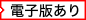•確率理論：科学の論理Probability Theory : The Logic of Science

アメリカ（US）から取り寄せる

• 提携先の海外書籍取次会社に在庫がございます。通常3週間で発送いたします。
重要ご説明事項
1. 納期遅延や、ご入手不能となる場合が若干ございます。
2. 複数冊ご注文の場合、分割発送となる場合がございます。
3. 美品のご指定は承りかねます。

イギリス（GB）から取り寄せる

• 提携先の海外書籍取次会社に在庫がございます。通常約2週間で発送いたします。
重要ご説明事項
1. 納期遅延や、ご入手不能となる場合が若干ございます。
2. 複数冊ご注文の場合、分割発送となる場合がございます。
3. 美品のご指定は承りかねます。
• 【重要：入荷遅延について】
各国での新型コロナウィルス感染拡大により、洋書・洋古書の入荷が不安定になっています。
海外からのお取り寄せの場合、弊社サイト内で表示している標準的な納期よりもお届けまでに日数がかかる見込みでございます。
申し訳ございませんが、あらかじめご了承くださいますようお願い申し上げます。

• 製本 Hardcover:ハードカバー版／ページ数 727 p.
• 言語 ENG
• 商品コード 9780521592710
• DDC分類 519.2

基本説明

Dispels the imaginary distinction between probability theory and statistical inference, leaving a logical unity and simplicity, which provides greater technical power and flexibility in applications.

Full Description

The standard rules of probability can be interpreted as uniquely valid principles in logic. In this book, E. T. Jaynes dispels the imaginary distinction between 'probability theory' and 'statistical inference', leaving a logical unity and simplicity, which provides greater technical power and flexibility in applications. This book goes beyond the conventional mathematics of probability theory, viewing the subject in a wider context. New results are discussed, along with applications of probability theory to a wide variety of problems in physics, mathematics, economics, chemistry and biology. It contains many exercises and problems, and is suitable for use as a textbook on graduate level courses involving data analysis. The material is aimed at readers who are already familiar with applied mathematics at an advanced undergraduate level or higher. The book will be of interest to scientists working in any area where inference from incomplete information is necessary.

Foreword
Preface
Part I. Principles and Elementary Applications:
1. Plausible reasoning
2. The quantitative rules
3. Elementary sampling theory
4. Elementary hypothesis testing
5. Queer uses for probability theory
6. Elementary parameter estimation
7. The central, Gaussian or normal distribution
8. Sufficiency, ancillarity, and all that
9. Repetitive experiments, probability and
frequency
10. Physics of 'random experiments'
Part II. Advanced Applications: 11. Discrete
prior probabilities, the entropy principle
12. Ignorance priors and transformation groups
13. Decision theory: historical background
14. Simple applications of decision theory